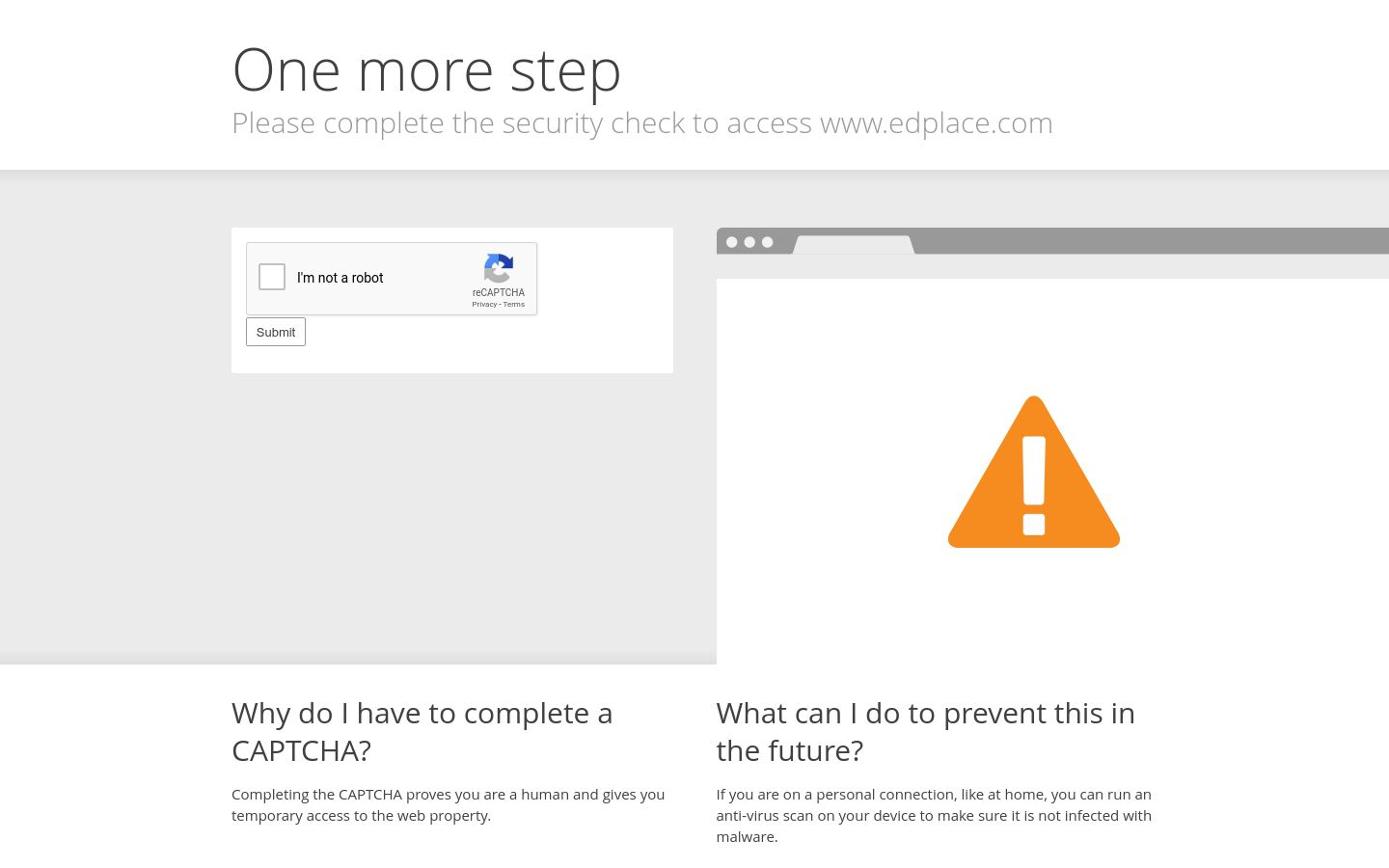# Geometry: Angles in a Triangle

This worksheet is about calculating the third angle in a triangle.Key stage:  KS 2

Curriculum topic:   Maths and Numerical Reasoning

Curriculum subtopic:   2D Shapes: Circles, Angles and Bearings

Difficulty level:### QUESTION 1 of 10Remember

The angles in any triangle add to 180°

So the missing angle = 180° - 54° - 64° = 62°

Two of the angles in a triangle are 77º and  66º.

Work out the size of the third angle in degrees.

(Just write the number)

Two of the angles in a triangle are 75º and  65º.

Work out the size of the third angle in degrees.

(Just write the number)

Two of the angles in a triangle are 68º and  67º.

Work out the size of the third angle in degrees.

(Just write the number)

Two of the angles in a triangle are 25º and  42º.

Work out the size of the third angle in degrees.

(Just write the number)

Two of the angles in a triangle are 25º and  25º.

Work out the size of the third angle in degrees.

(Just write the number)

Two of the angles in a triangle are 130º and  25º.

Work out the size of the third angle in degrees.

(Just write the number)

Two of the angles in a triangle are 101º and  25º.

Work out the size of the third angle in degrees.

(Just write the number)

Two of the angles in a triangle are 131º and  25º.

Work out the size of the third angle in degrees.

(Just write the number)

Two of the angles in a triangle are 97º and  36º.

Work out the size of the third angle in degrees.

(Just write the number)

Two of the angles in a triangle are 170º and  .

Work out the size of the third angle in degrees.

(Just write the number)

• Question 1

Two of the angles in a triangle are 77º and  66º.

Work out the size of the third angle in degrees.

(Just write the number)

37
EDDIE SAYS
180 - 77 - 66
• Question 2

Two of the angles in a triangle are 75º and  65º.

Work out the size of the third angle in degrees.

(Just write the number)

40
EDDIE SAYS
180 - 75 - 65
• Question 3

Two of the angles in a triangle are 68º and  67º.

Work out the size of the third angle in degrees.

(Just write the number)

45
EDDIE SAYS
180 - 68 - 67
• Question 4

Two of the angles in a triangle are 25º and  42º.

Work out the size of the third angle in degrees.

(Just write the number)

113
• Question 5

Two of the angles in a triangle are 25º and  25º.

Work out the size of the third angle in degrees.

(Just write the number)

130
• Question 6

Two of the angles in a triangle are 130º and  25º.

Work out the size of the third angle in degrees.

(Just write the number)

25
• Question 7

Two of the angles in a triangle are 101º and  25º.

Work out the size of the third angle in degrees.

(Just write the number)

54
• Question 8

Two of the angles in a triangle are 131º and  25º.

Work out the size of the third angle in degrees.

(Just write the number)

24
• Question 9

Two of the angles in a triangle are 97º and  36º.

Work out the size of the third angle in degrees.

(Just write the number)

47
• Question 10

Two of the angles in a triangle are 170º and  .

Work out the size of the third angle in degrees.

(Just write the number)